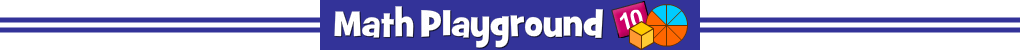There are 8 wild animals hiding in the wall.
How many steps to uncover them all?
Practice number names. Three levels to try.
Untamed Number Names - Learning Connections
Essential Skills
Mental Math - identify number names

Common Core Connection for Grades 1 and 2
Read and write numerals and represent a number of objects with a written numeral.
Understand that the 2 digits of a 2-digit number represent tens and ones.
Understand that the 3 digits of a 3-digit number represent hundreds, tens, and ones.
More Math Games to Play